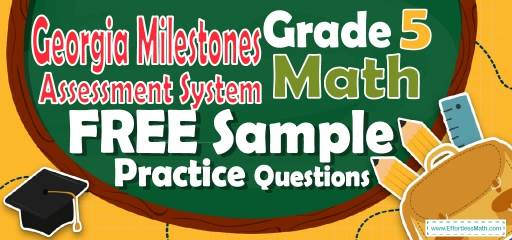# 5th Grade Georgia Milestones Assessment System Math FREE Sample Practice QuestionsPreparing your student for the 5th Grade Georgia Milestones Assessment System Math test? To succeed on the Georgia Milestones Assessment System Math test, students need to practice as many real Georgia Milestones Assessment System Math questions as possible.  There’s nothing like working on Georgia Milestones Assessment System Math sample questions to measure your student’s exam readiness and put him/her more at ease when taking the 5th Grade Georgia Milestones Assessment System Math test. The sample math questions you’ll find here are brief samples designed to give students the insights they need to be as prepared as possible for their 5th Grade Georgia Milestones Assessment System Math test.

Check out our sample 5th Grade Georgia Milestones Assessment System Math practice questions to find out what areas your student needs to practice more before taking the 5th Grade Georgia Milestones Assessment System Math test!

Start preparing your student for the 2022 Georgia Milestones Assessment System Math test with our free sample practice questions. Also, make sure to follow some of the related links at the bottom of this post to get a better idea of what kind of mathematics questions students need to practice.

## 10 Sample 5th Grade Georgia Milestones Assessment System Math Practice Questions

1- What is the volume of this box?
$$\img {https://appmanager.effortlessmath.com/public/images/questions/test5.staar5.222.JPG }$$

A. 24 cm$$^3$$

B. 32 cm$$^3$$

C. 162 cm$$^3$$

D. 192 cm$$^3$$

2- How many square feet of tile is needed for 18 feet to 18 feet room?

A. 72 Square Feet

B. 108 Square Feet

C. 216 Square Feet

D. 324 Square Feet

3- Of the 2,400 videos available for rent at a certain video store, 600 are comedies. What percent of the videos are comedies?

A. $$18\frac{1}{2}\%$$

B. $$20\%$$

C. $$22\%$$

D. $$25\%$$

4- How many $$3 × 3$$ squares can fit inside a rectangle with a height of 54 and width of 12?

A. 72

B. 62

C. 50

D. 44

5- ABC Corporation earned only $200,000 during the previous year, two-thirds only of the management’s predicted income. How much earning did the management predict? A.$20,000

B. $30,000 C.$300,000

D. \$340,000

6- William keeps track of the length of each fish that he catches. Following are the lengths in inches of the fish that he caught one day: $$13, 14, 9, 11, 9, 10, 18$$
What is the median fish length that William caught that day?

A. 11 Inches

B. 9 Inches

C. 12 Inches

D. 13 Inches

7- Solve: $$9 + [8 × 5] ÷ 2 =$$ ? ________

8- What is the median of these numbers? $$4, 9, 13, 8, 15, 18, 5$$

A. 8

B. 9

C. 13

D. 15

9- The area of the base of the following cylinder is 50 square inches and its height is 10 inches. What is the volume of the cylinder? ________

10- A rope 13 yards long is cut into 4 equal parts. Which expression does NOT equal the length of each part?

A. $$13 ÷ 4$$

B. $$\frac{13}{4}$$

C. $$4 ÷ 13$$

D. $$4\sqrt{13}$$

## Best 5th Grade Georgia Milestones Assessment System Math Exercise Resource for 2022

1- D
Use volume of cube formula.
Voluem$$=$$ length $$×$$ width$$×$$ height$$⇒ V= 6 × 4 × 8 ⇒ V=192 \space$$$$cm^3$$

2- D
Find the area of the room which is a square. Use area of square formula.
$$S= a^2 ⇒ S= 18$$ feet $$× 18$$ feet $$= 324$$ square feet

3- D
Use percent formula:
part$$=\frac{percent}{100}×$$whole
$$600 =\frac{percent}{100}× 2400⇒ 600=$$ percent $$× 24 ⇒$$percent$$=25$$

4- A
Use area of rectangle formula.
$$A= a × b ⇒ A= 54 × 12 ⇒ A= 648$$
Divide the area by 9 ($$3 × 3 = 9$$ squares) to find the number of squares needed. $$648 ÷ 9 = 72$$

5- C
ABC Corporation’s income $$= \frac{2}{3}$$ management’s predicted income.
$$200,000 = \frac{2}{3}$$ management’s predicted income
management’s predicted income $$= 200,000×\frac{3}{2} =300,000$$

6- A
Write the numbers in order:
$$9, 9, 10, 11, 13, 14, 18$$
Median is the number in the middle. Therefore, the median is 11.

7- 29
Use PEMDAS (order of operation):
$$9 + [8 × 5] ÷ 2 = 9 + (40) ÷ 2 = 9 + (40 ÷ 2) = 29$$

8- B
Write the numbers in order:
$$4, 5, 8, 9, 13, 15, 18$$
Median is the number in the middle. Therefore, the median is 9.

9- 500
Use volume of cylinder formula.
Voluem$$=$$ base $$×$$ heigth $$⇒ V=50 × 10 ⇒ V=500$$

10- C
13 yards long rope is cut into 4 equal parts. Therefore, 13 should be divided by 4. Only option C is NOT 13 divided by 4. (It is 4 divided by 13)

Looking for the best resource to help you succeed on the 5th Grade Georgia Milestones Assessment System Math test?